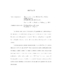## Measurement of the Electric Form Factor of the Neutron at High Momentum Transfer2009
##### Authors
Miller, Jonathan Andrew
The electric form factor of the neutron, $G_{E}^{n}$, provides key understanding of the structure of one of the basic building blocks of visible matter in the universe. Recent interest in this quantity is the result of the improved quality of data provided by double polarization experiments, which have substantially improved in the last decade. This thesis presents precision measurements of $G_{E}^{n}$ by the E02-013 collaboration at $Q^{2}$ of 1.7, 2.5, and 3.5 GeV$^{2}$. This measurement used a double polarization technique, a highly polarized $^{3}$He target, a polarized electron beam, a large acceptance spectrometer to detect the scattered electrons, and a large neutron detector to detect the recoiling hadrons in the reaction $^{3}\vec{\mathrm{He}}(\vec{e},e'n)$. These measurements will be compared to a variety of models of the nucleon's internal structure, as well as used to extract individual contributions of the up and down quarks to the nucleon form factors.